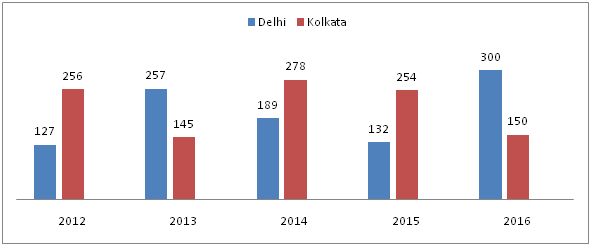# IBPS SO / NIACL AO Prelims – Quantitative Aptitude Questions Day- 61

Dear Readers, Bank Exam Race for the Year 2018 is already started, To enrich your preparation here we have providing new series of Practice Questions on Quantitative Aptitude – Section. Candidates those who are preparing for IBPS SO / NIACL AO Prelims 2018 Exams can practice these questions daily and make your preparation effective.

[WpProQuiz 4690]

Directions (Q. 1 – 5): What approximate value should come in place of question mark (?) in the following questions?

1) 39. 98 % of 4303 + 58.79 % of 5003 =? – 3867.87

a) 9150

b) 7560

c) 8540

d) 6770

e) 5680

2) √11450 × √6240 – (53.96)2 = √? + (74.01)2

a) 3364

b) 3136

c) 3969

d) 3721

e) 4225

3) ? = (9786 + 549) ÷ 64.89 + (36.11)2 + (1/4) of 1679

a) 1650

b) 1875

c) 2135

d) 2470

e) 2815

4) 18 (½) % of 9800 + 23 (1/3) % of 8700 = (5/9) of 809 +?

a) 2900

b) 3050

c) 3415

d) 2625

e) 4095

5) (5/7) of 341 + (2/3) of (7/15) of 1889 =? % of 700

a) 150

b) 90

c) 120

d) 210

e) 250

Directions (Q. 6 – 10): Study the following information carefully and answer the given questions:

The following bar graph shows the number of Pan Card holders (in thousands) from Delhi and Kolkata in different years.6) Find the difference between the total number of pan card holders from Delhi in the year 2013 and 2014 to that of total number of pan card holders from Kolkata in the year 2012 and 2016 together?

a) 36000

b) 40000

c) 48000

d) 52000

e) None of these

7) Total number of pan card holders from Delhi in the year 2016 is what percentage of total number of pan card holders from Kolkata in all the given years together?

a) 31.5 %

b) 42.9 %

c) 27.7 %

d) 39.3 %

e) None of these

8) If in the year 2017, total number of pan card holders from Delhi and Kolkata together is increased by 15 % and 30 % respectively while comparing with previous year, then find the ratio between the total number of pan card holders from Delhi and Kolkata in the year 2017?

a) 16: 8

b) 35: 26

c) 28: 17

d) 23: 13

e) None of these

9) The average number of pan card holders from Delhi in the year 2012, 2015 and 2016 together is approximately what percentage more/less than the average number of pan card holders from Kolkata in the same year?

a) 15 % less

b) 15 % more

c) 28 % less

d) 28 % more

e) 37 % more

10) Total number of pan card holders in Kolkata in the year 2015 is 5 % of the total population in that year. Find the total population in the year 2015?

a) 4560000

b) 6420000

c) 5080000

d) 6090000

e) None of these

Direction (1-5) :

40 % of 4300 + 59 % of 5000 = x – 3868

(40/100)*4300 + (59/100)*5000 + 3868 = x

X = 1720 + 2950 + 3868

X = 8538 = 8540

√11449 × √6241 – (53.96)2 = √? + (74.01)2

107 * 79 – 542 – 742 = √x

8453 – 2916 – 5476 = √x

61 = √x

X = 612 = 3721

X = (10335/65) + 1296 + (1/4)*1680

X = 159 + 1296 + 420

X = 1875

19 % of 9800 + 23 % of 8700 = (5/9) of 810 + x

(19/100)*9800 + (23/100)*8700 = (5/9)*810 + x

1862 + 2001 – 450 = x

X = 3413 = 3415

(5/7) of 343 + (2/3) of (7/15) of 1890 = x % of 700

(5/7) * 343 + (2/3) * (7/15) * 1890 = (x/100)*700

245 + 588 = 7x

833 = 7x

X = 833/7

X = 119 = 120

Direction (6-10) :

Total number of pan card holders from Delhi in the year 2013 and 2014

= > 257000 + 189000 = 446000

Total number of pan card holders from Kolkata in the year 2012 and 2016

= > 256000 + 150000 = 406000

Required difference = 446000 – 406000 = 40000

Total number of pan card holders from Delhi in the year 2016 = 300000

Total number of pan card holders from Kolkata in all the given years

= > (256 + 145 + 278 + 254 + 150)*1000 = 1083000

Required % = (300000/1083000)*100 = 30000/1083 = 27.7 %

In the year 2017,

Total number of pan card holders from Delhi = 300*(115/100) = 345

Total number of pan card holders from Kolkata = 150*(130/100) = 195

Required ratio = 345: 195 = 23: 13

The average number of pan card holders from Delhi in the year 2012, 2015 and 2016 together

= > (127000 + 132000 + 300000)/3 = 559000/3

The average number of pan card holders from Kolkata in the year 2012, 2015 and 2016 together

= > (256000 + 254000 + 150000)/3 = 660000/3

Required % = {[660000/3 – 559000/3] / 660000/3}*100

= > (101000/660000)*100

= >15.30 % = 15 % less

Total number of pan card holders in Kolkata in the year 2015 = 254000

254000 = 5 % of total population

Total population = 254000*(100/5) = 5080000